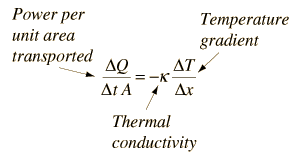# Thermal Conductivity

In physics, thermal conductivity(often denoted k, λ, or κ) is the property of a material to conduct heat. It is evaluated primarily in terms of Fourier’s Law for heat conduction.

Heat transfer occurs at a lower rate across materials of low thermal conductivity than across materials of high thermal conductivity. Correspondingly, materials of high thermal conductivity are widely used in heat sink applications and materials of low thermal conductivity are used as thermal insulation. For materials, it may depend on temperature. The reciprocal of thermal conductivity is called thermal resistivity.

Heat transfer by conduction involves the transfer of energy within a material without any motion of the material as a whole. The rate of heat transfer depends upon the temperature gradient and the thermal conductivity of the material. It is a reasonably straightforward concept when you are discussing heat loss through the walls of your house, and you can find tables which characterize the building materials and allow you to make reasonable calculations.

More fundamental questions arise when you examine the reasons for wide variations in thermal conductivity. Gases transfer heat by direct collisions between molecules, and as would be expected, their thermal conductivity is low compared to most solids since they are dilute media. Non-metallic solids transfer heat by lattice vibrations so that there is no net motion of the media as the energy propagates through. Such heat transfer is often described in terms of “phonons”, quanta of lattice vibrations. Metals are much better thermal conductors than non-metals because the same mobile electrons which participate in electrical conduction also take part in the transfer of heat.

### The Concept of Thermal Conductivity:

It can be thought of as the container for the medium-dependent properties which relate the rate of heat loss per unit area to the rate of change of temperature.It (λ with the unit W/(m•K)) describes the transport of energy – in the form of heat – through a body of mass as the result of a temperature gradient (see fig. 1). According to the second law of thermodynamics, heat always flows in the direction of the lower temperature.

The relationship between transported heat per unit of time (dQ/dt or heat flow Q) and the temperature gradient (ΔT/Δx) through Area A (the area through which the heat is flowing perpendicularly at a steady rate) is described by the thermal conductivity equation.